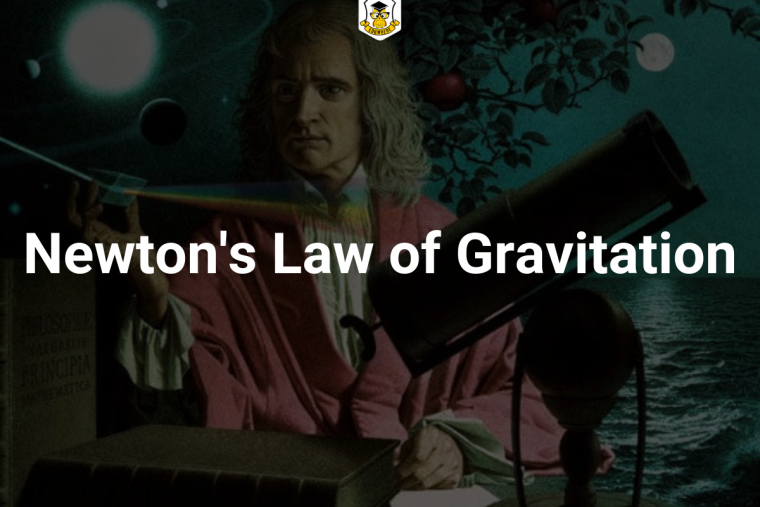## Eduwhere Concept: Newton's Law of GravitationEven if we all have learnt Newton’s law of gravitation in school and college, it has been a part of our studies after that too.

Here is a quick revision for Newton’s law of gravitation for your upcoming competitive examination.

Statement:

#### Newton’s Law of Gravitation

Gravitational force (F) between two bodies is directly proportional to the product of masses and inversely proportional to the square of the distance between them.

#### Explanation:

Let m1 and m2 be the masses of two bodies and r be the separation between them.

F ∝ m1m2 / r2

Here, G is gravitational constant.

Value of G is (6.67 × 10-11 N m2 kg-2))

Acceleration due to gravity

For a body falling freely under gravity, the acceleration in the body is called acceleration due to gravity.

Relationship between g and G is given by

g = GMe /Re = 4/3 πGReΡ

where G = gravitational constant, Me and Re be the mass and radius of the earth.

Characteristics of gravitational force:

• It is always attractive.
• It is independent of the medium.
• It is a conservative and central force.
• It holds good over a wide range of distance.

1

954